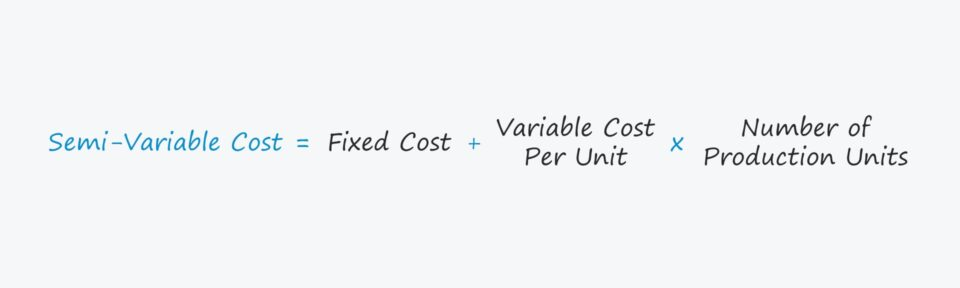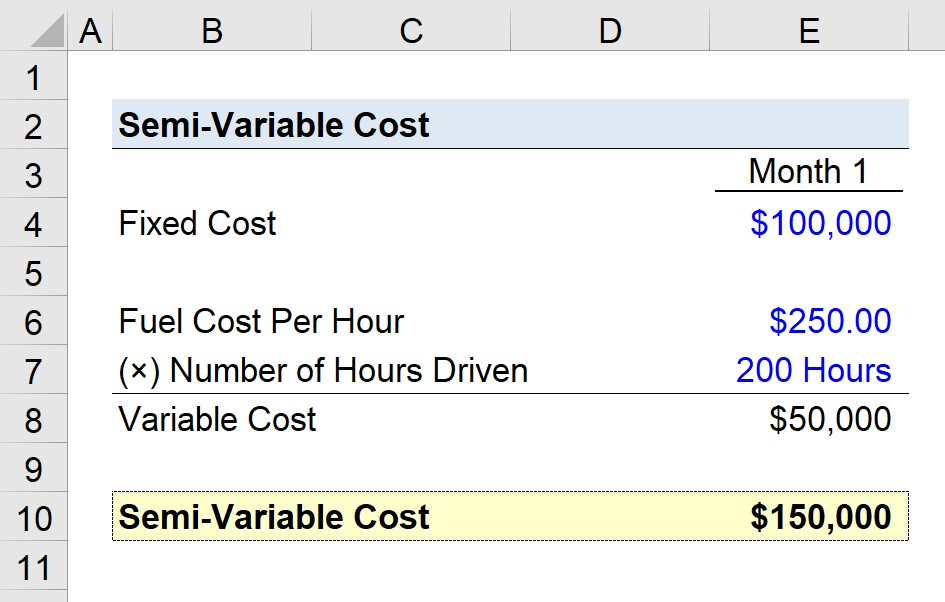Welcome to Wall Street Prep! Use code at checkout for 15% off.# Semi-Variable Cost

Guide to Understanding Semi-Variable Costs## How to Calculate Semi-Variable Costs?

A semi-variable cost consists of a fixed component as well as a variable component that can cause the total cost to increase or decrease based on the circumstances on hand.

Conceptually, semi-variable costs are a hybrid between fixed and variable costs.

• Fixed Costs → Output-independent costs with a dollar amount incurred that remains constant regardless of a company’s production volume.
• Variable Costs → Output-dependent costs that are a direct function of the production volume and thereby fluctuate each period based on the stated output level.

Semi-variable costs blend features of fixed and variable costs and represent a nuance to the generalized fixed vs. variable cost categorization.

Given how the dollar value of fixed costs remains unchanged whether a company outperforms (or underperforms), these sorts of costs are much easier to predict and forecast for budgeting purposes.

On the other hand, variable costs are subject to fluctuations based on the current period’s production output, while variable costs can either increase or decrease depending on output in a specific period, making them more challenging to predict.

Certain costs, however, cannot be classified as purely fixed or variable costs, as they are a “blend” of the two types, i.e. a semi-variable cost.

## Semi-Variable Cost Formula

The formula for calculating the semi-variable cost is as follows.

Semi-Variable Cost = Fixed Cost + (Variable Cost × Number of Production Units)

The number of production units is the fluctuating volume metric that determines the variable component of the cost, e.g. number of miles driven or the number of units produced.

## Semi-Variable Cost Calculator

We’ll now move to a modeling exercise, which you can access by filling out the form below.Submitting...

## Semi-Variable Cost Example Calculation

Suppose a trucking company is attempting to estimate its semi-variable costs for its most recent month, Month 1.

The company incurred \$100,000 in fixed costs related to rental costs and insurance, among others.

• Fixed Costs = \$100,000

The \$100k represents the fixed component, so we’ll now calculate the variable component, which is the fuel cost in our hypothetical scenario.

The fuel cost per hour is \$250.00, while the number of hours driven in Month 1 is 200 hours.

• Fuel Cost Per Hour = \$250.00
• Number of Hours Driven = 200 Hours

The product of the fuel cost per hour and the number of hours driven (\$50,000) is the trucking company’s variable cost component.

• Variable Cost = \$250.00 × 200 = \$50,000

Our total is the sum of the fixed and variable cost components, which is \$150,000.

• Semi-Variable Cost = \$100,000 + \$50,000 = \$150,000Step-by-Step Online Course

### Everything You Need To Master Financial Modeling

Enroll in The Premium Package: Learn Financial Statement Modeling, DCF, M&A, LBO and Comps. The same training program used at top investment banks.